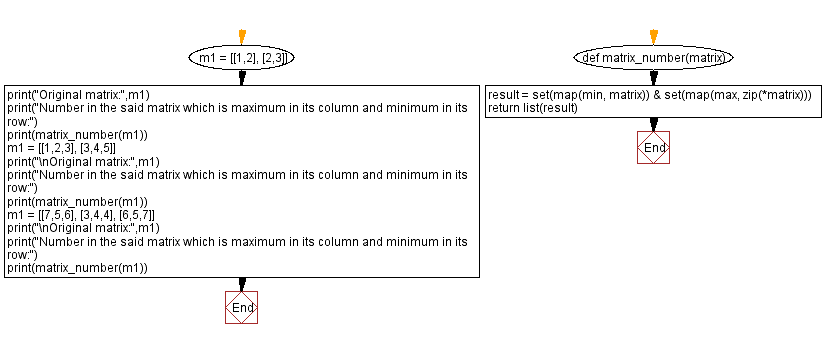﻿ Python: Find a number in a given matrix which is maximum in its column and minimum in its row - w3resource# Python: Find a number in a given matrix which is maximum in its column and minimum in its row

## Python Basic - 1: Exercise-104 with Solution

Write a Python program to find a number in a given matrix, which is maximum in its column and minimum in its row.

Sample Solution:

Python Code:

``````# Number in a Matrix: Maximum in its column and minimum in its row.
def matrix_number(matrix):
result = set(map(min, matrix)) & set(map(max, zip(*matrix)))
return list(result)
m1 = [[1,2], [2,3]]
print("Original matrix:",m1)
print("Number in the said matrix which is maximum in its column and minimum in its row:")
print(matrix_number(m1))
m1 = [[1,2,3], [3,4,5]]
print("\nOriginal matrix:",m1)
print("Number in the said matrix which is maximum in its column and minimum in its row:")
print(matrix_number(m1))
m1 = [[7,5,6], [3,4,4], [6,5,7]]
print("\nOriginal matrix:",m1)
print("Number in the said matrix which is maximum in its column and minimum in its row:")
print(matrix_number(m1))
``````

Sample Output:

```Original matrix: [[1, 2], [2, 3]]
Number in the said matrix which is maximum in its column and minimum in its row:


Original matrix: [[1, 2, 3], [3, 4, 5]]
Number in the said matrix which is maximum in its column and minimum in its row:


Original matrix: [[7, 5, 6], [3, 4, 4], [6, 5, 7]]
Number in the said matrix which is maximum in its column and minimum in its row:

```

Flowchart:Python Code Editor:

Have another way to solve this solution? Contribute your code (and comments) through Disqus.

What is the difficulty level of this exercise?

Test your Programming skills with w3resource's quiz.

﻿

## Python: Tips of the Day

What is the difference between Python's list methods append and extend?

append: Appends object at the end.

```x = [1, 2, 3]
x.append([4, 5])
print (x)
```

Output:

```[1, 2, 3, [4, 5]]
```

extend: Extends list by appending elements from the iterable.

```x = [1, 2, 3]
x.extend([4, 5])
print (x)
```

Output:

```[1, 2, 3, 4, 5]
```

Ref: https://bit.ly/2AZ6ZFq